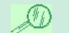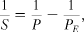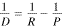MORE PRECISELY 9-1 Synodic Periods and Solar Days Recall from Chapter 1 that a body's sidereal orbital (or rotational) period is the time taken for it to complete one orbit (or rotation) relative to the "fixed" stars.(Sec. 1.3) The synodic orbital period is defined to be the time taken for the body to return to the same configuration relative to the Sun, taking Earth's own motion into account.(Sec. 1.4) Examples are the time from one full Moon to the next, or between successive inferior conjunctions (times of closest approach) of Venus. A closely related concept is the solar day, the time from noon to noon, which in general differs from the sidereal day because of a planet's motion around the Sun.(Sec. 1.3) In the case of Earth, the difference between a sidereal and a solar day is relatively small, as is the difference between a sidereal and a synodic month. However, as we have seen, Mercury's sidereal and solar days differ significantly, as do the sidereal and synodic periods of all the planets. Let's take a moment to look at this topic in a little more detail. Calculating the synodic period is easy. Denoting Earth's sidereal orbital period (365.26 solar days) by PEand that of the body in question by P, we see then that the angular speeds of Earth and the other body (in degrees per day, say) are, respectively, 360°/PE and 360°/P, since there are 360 degrees in one complete revolution. Thus, the rate at which the body outruns Earth is 360°/P—360°/PE. The body's synodic period S is by definition the time taken for the body to "lap" Earth (that is, outstrip it by 360°), so it follows thatwhere S is positive for the interior planets Mercury and Venus (having P less than PE) and negative for the exterior planets Mars, Jupiter, and so on (meaning that Earth overtakes them). For example, the Moon has P = 27.3 days, so its synodic period is S = 29.5 days; for Venus, P = 224.7 days, yielding a synodic period S = 583.9 days. Similar reasoning applied to the competition between the planet's sidereal rotation period R and its sidereal orbital period P leads to the following expression for the planetary solar day D:Earth has R = 0.9973 solar days and P = PE = 365.26 solar days, which gives D = 1 solar day, as expected. The difference between Earth's solar and sidereal days is small because Earth rotates on its axis much more rapidly than it revolves around the Sun. For Mercury and Venus, however, the rotation and revolution periods are comparable and the difference between solar and sidereal days is much greater. Using the data from The Mercury Data box, we find, for Mercury, R = 58.6 (Earth) solar days, P = 88.0 solar days, so D = 176.0 solar days, or two sidereal years.(Sec. 8.2) Venus has R = -243.0 solar days and P = 224.7 solar days, so D = -116.7 solar days (the negative signs, as usual, indicate retrograde rotation). The extreme example would be a planet in a synchronous orbit around the Sun (or the Moon orbiting Earth), with R = P. In that case, D would be infinite—the Sun would never set! Sidereal periods are "physical," in the sense that they are the quantities to which the laws of Kepler and Newton refer. However, from the point of view of timekeeping, skywatching, or scheduling a mission to another planet, synodic periods are in many ways just as important.